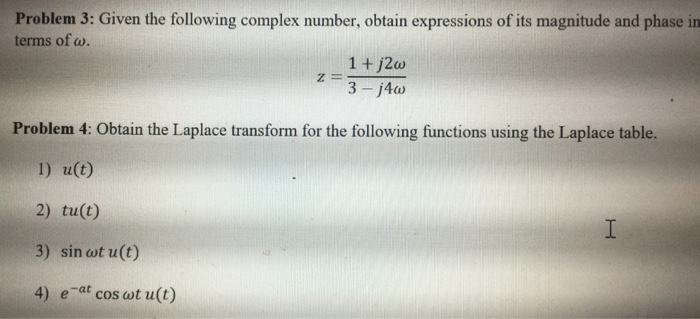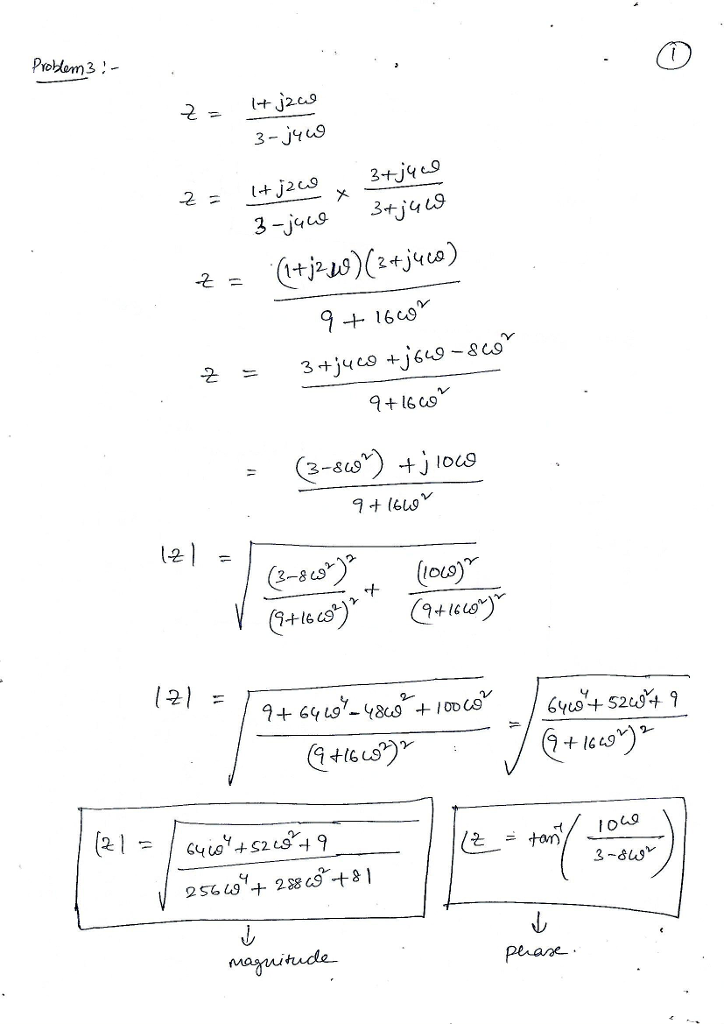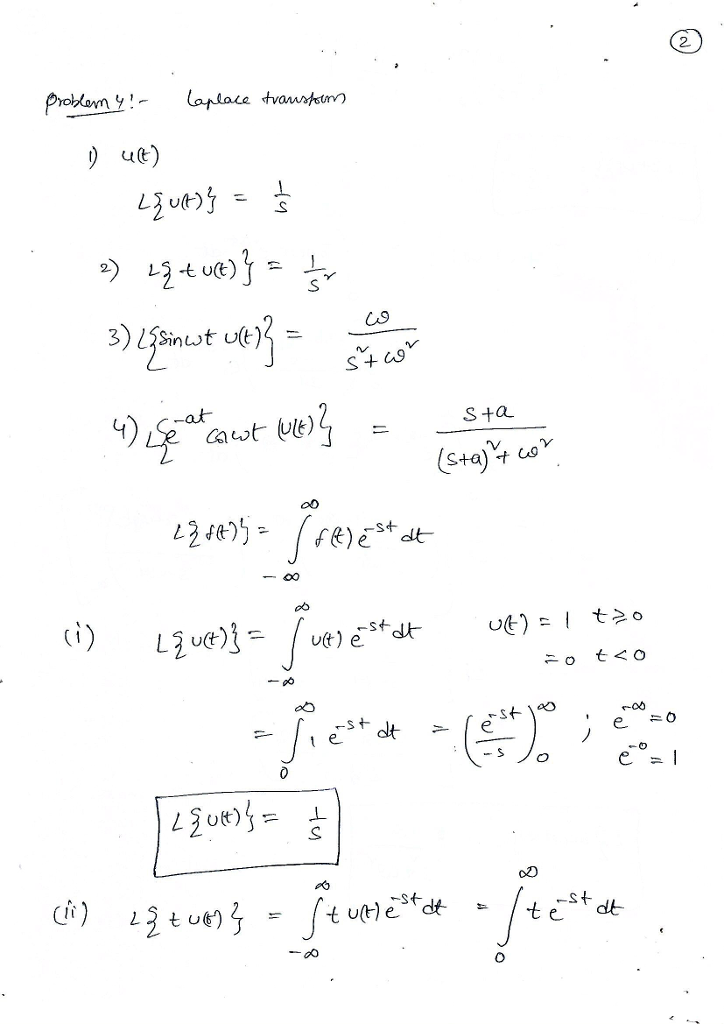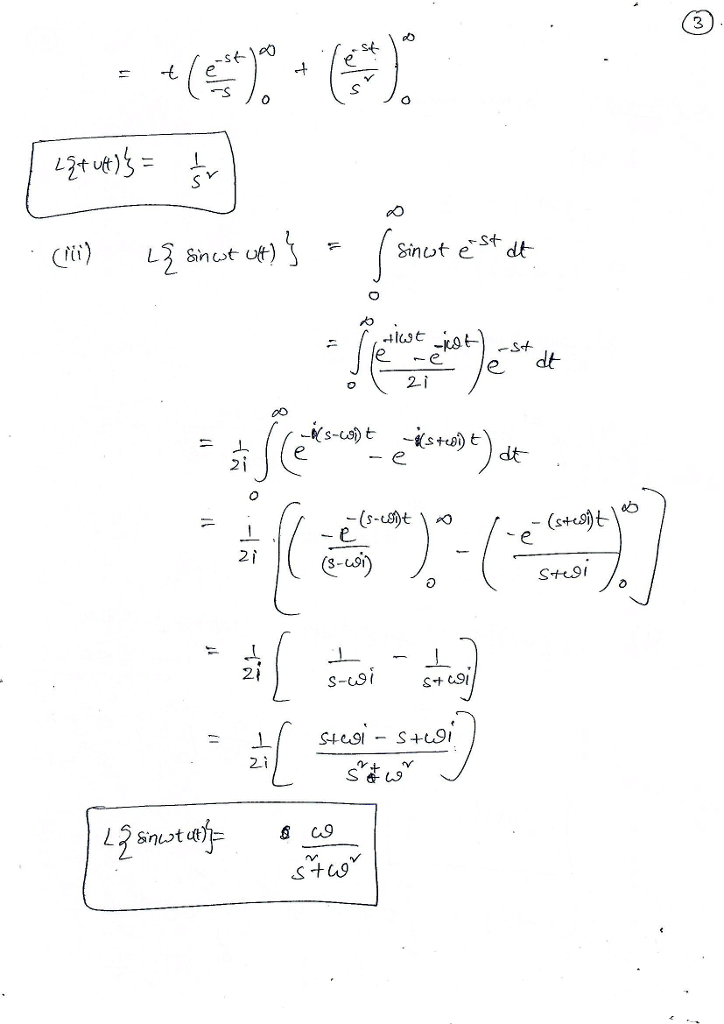# Homework Solution: Given the following complex number, obtain expressions of its magnitude and phase in terms of omega. z = 1 + j2 omega/3 – j4 omega Obtain the Lap…Given the following complex number, obtain expressions of its magnitude and phase in terms of omega. z = 1 + j2 omega/3 - j4 omega Obtain the Laplace transform for the following functions using the Laplace table. 1) u(t) 2) tu(t) 3) sin omega t u(t) 4) e^-at cos omega t u(t)